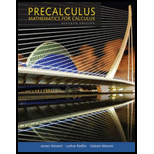Chapter 7, Problem 58REPrecalculus: Mathematics for Calcu...

7th Edition
James Stewart + 2 others
ISBN: 9781305071759

Solutions

Chapter
SectionPrecalculus: Mathematics for Calcu...

7th Edition
James Stewart + 2 others
ISBN: 9781305071759
Textbook Problem

Value of Expressions Find the exact value of the expression.58. cos 67.5° + cos 22.5°

To determine

To find: The exact value of the expression cos67.5°+cos22.5° .

Explanation

Formula used:

Trigonometric identity: cosA+cosB=2cos(A+B2)cos(AB2) (1)

Calculation:

The expression cos67.5°+cos22.5° rewritten as follows.

From the equation (1),

Let A=67.5 and B=22.5 .

cos67.5°+cos22.5°=2cos(67.5°+22.5°2)cos(67.5°22.5°2)=2cos(90°2)cos(45°2)=2cos45°cos22.5°=2cos45°[1+cos45°2]

On further simplification and obtain the value of expression,

cos67

Still sussing out bartleby?

Check out a sample textbook solution.

See a sample solution

The Solution to Your Study Problems

Bartleby provides explanations to thousands of textbook problems written by our experts, many with advanced degrees!

Get Started